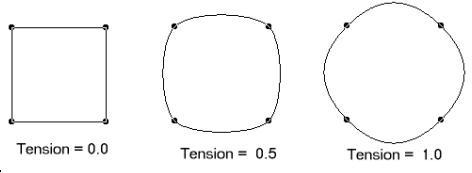﻿ Tension Property (AnnCurveObject) | Leadtools.Annotations.Engine | Raster, Medical, Document Help
←Select platform
In This Topic ▼

# Tension Property

Summary
Gets or sets a value that specifies the amount that this AnnCurveObject curve bends between control points.
Syntax
C#
Objective-C
C++
Java
``public double Tension { get; set; } ``
``@property (nonatomic, assign) double tension; ``
````public double getTension() `
`public void setTension(double tension) ````
````public:  `
`   property double Tension `
`   { `
`      double get() `
`      void set(double value) `
`   } ````

#### Property Value

A value that specifies the amount that this AnnCurveObject curve bends between control points.

Remarks

Valid values are between 0.0 and 1.0, where 0.0 indicates straight lines, and 1.0 indicates lines with the highest curvature. A typical value is 0.5. These values for Tension for a Closed Curve object are shown in the following bitmap:Requirements